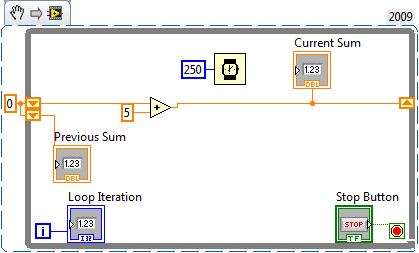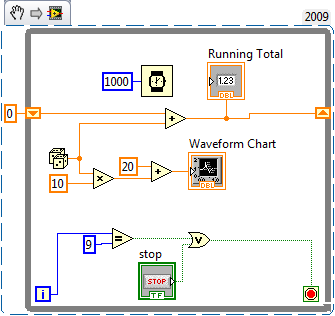# Curriculum and Labs for Engineering Education

cancel
Showing results for
Did you mean:

## Unit 2 - Fundamentals: Lesson 3

Introduction

In this lesson we are going to be talking about shift registers. Shift registers are a feature of while and for loops which allw them to maintain memory.

Lesson Video

Example SnippetPractice Problem

Procedure: Create the required VI(s) to achieve the following tasks.

Requirements:

1. Modify the Practice Problem from Unit 2 Lesson 2 so that the total sum of all the random numbers generated is displayed.

Practice SolutionNext Step

Unit 2 - Fundamentals: Lesson 4